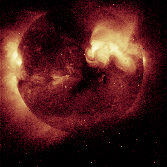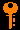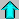# Lecture 10 - Radiative Transfer (2/16/99)Stat Mech --- | --- Stellar Structure IReading:
Chapter 8-7, 10-2, 13-1 (ZG4)

Notes:
pages 38 - 42

This is an X-ray image of the Sun obtained on February 21, 1994. The brighter regions are sources of increased X-ray emissions. (Courtesy Calvin J. Hamilton, and Yohkoh)
Key Question:## Investigations:

1. Radiative Transfer II - Opacity and Optical Depth
• How does the absorption depend upon the gas density?
• How does the absorption depend upon the incident intensity?
• How does the absorption depend upon the layer thickness?
• What is the opacity of a gas?
• What is the optical depth of an absorbing medium?
• How do you solve the differential equation for pure absorption?
• Why is the solution to the radiative transfer equation an exponential in the optical depth?
• What are the implications of this for the case of observed photons from the solar photosphere?
• How deep is the tau=1 layer in the solar photosphere?
2. The Plane-Parallel Atmosphere
• How does the radiative transfer equation scale with inclination angle?
• What is the airmass?
• In what limit can observations through the Earth's atmosphere be approximated as absorption in a plane-parallel medium?
• How can observations at different angles be used to solve for the zenith optical depth and the incident intensity?
• Why is this important for observational astronomy?
3. Radiative Transfer III - Emission + Absorption
• How does the emission depend upon the density and thickness?
• What is the source function?
• What is the radiative transfer equation?
• Where in the radiative transfer equation is all the physics contained?
• How does the source function "drag" the intensity toward its value?
• What is the solution to the radiative transfer equation for a uniform intensity medium?
• What is the source function for a medium in thermodynamic equilibrium?
• What is the solution for a constant source function?
• How fast is the incident intensity replaced by the thermal emission of the medium?
4. Opacity in the Solar Photosphere
• How do we know that the solar photosphere is opaque at optical wavelengths?
• How can the photosphere be opaque at the low densities and temperatures prevalent there?
• What is the primary opacity agent in the solar photosphere?
• What is the H- ion?
• What is the ionization potential of H- compared with that of H?
• What is meant by a bound-free transition?
• What is a bound-bound transition?
• What is free-free absorption and emission?
• What is electron scattering?
• Upon what things do the opacity and source function in a stellar atmosphere depend?
5. Interlude - Iterative Solution of Equations
• How do you find the maximum of the blackbody function (Wien's Law)?
• How do you solve the Saha equation for the temperature at a fixed ionization fraction?
• How can you iteratively solve an equation x=f(x)?
• How is this related to root-finding?
• What determines whether this procedure converges?

## Iterative Solution of Numerical Problems

I mentioned in class that a wide range of numerical problems can be solved iteratively, often with just your calculator. These problems are of the type that can be put in the form

x = f(x)

and that starting with a guess x_1 you can iterate

xi+1 = f( xi )

to arrive at a stable solution. Note that there are often 2 or more ways to set up the equation, since by taking the inverse x = f-1(x) should work also (that isnt an exponent, but a functional inverse).Prev Lecture ---Next Lecture ---Astr12 Index ---Astr12 Home

smyers@nrao.edu Steven T. Myers